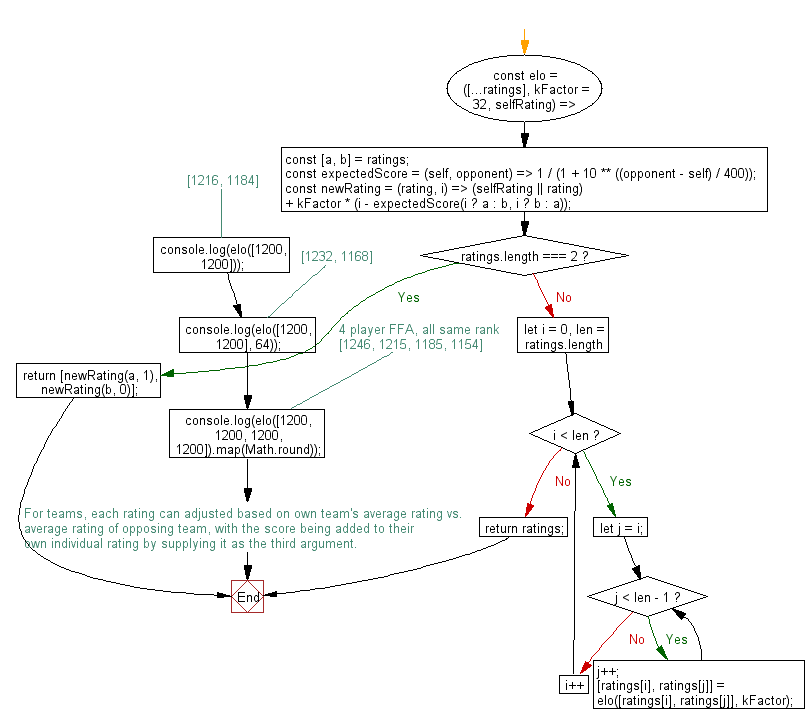# JavaScript: Compute the new ratings between two or more opponents using the Elo rating system

## JavaScript fundamental (ES6 Syntax): Exercise-75 with Solution

Write a JavaScript program to compute the new ratings between two or more opponents using the Elo rating system. It takes an array of pre-ratings and returns an array containing post-ratings. The array should be ordered from best performer to worst performer (winner -> loser).

Note: Use the exponent ** operator and math operators to compute the expected score (chance of winning). of each opponent and compute the new rating for each. Loop through the ratings, using each permutation to compute the post-Elo rating for each player in a pairwise fashion. Omit the second argument to use the default kFactor of 32.

Sample Solution:

JavaScript Code:

``````//#Source https://bit.ly/2neWfJ2
const elo = ([...ratings], kFactor = 32, selfRating) => {
const [a, b] = ratings;
const expectedScore = (self, opponent) => 1 / (1 + 10 ** ((opponent - self) / 400));
const newRating = (rating, i) =>
(selfRating || rating) + kFactor * (i - expectedScore(i ? a : b, i ? b : a));
if (ratings.length === 2) {
return [newRating(a, 1), newRating(b, 0)];
}
for (let i = 0, len = ratings.length; i < len; i++) {
let j = i;
while (j < len - 1) {
j++;
[ratings[i], ratings[j]] = elo([ratings[i], ratings[j]], kFactor);
}
}
return ratings;
};

console.log(elo([1200, 1200])); // [1216, 1184]
console.log(elo([1200, 1200], 64)); // [1232, 1168]
// 4 player FFA, all same rank
console.log(elo([1200, 1200, 1200, 1200]).map(Math.round)); // [1246, 1215, 1185, 1154]
/*
For teams, each rating can adjusted based on own team's average rating vs.
average rating of opposing team, with the score being added to their
own individual rating by supplying it as the third argument.
*/
```
```

Sample Output:

```[1216,1184]
[1232,1168]
[1246,1215,1185,1154]
```

Flowchart:Live Demo:

See the Pen javascript-basic-exercise-75-1 by w3resource (@w3resource) on CodePen.

Improve this sample solution and post your code through Disqus

What is the difficulty level of this exercise?

Test your Programming skills with w3resource's quiz.

﻿

## JavaScript: Tips of the Day

How to remove a specific item from an array?

Find the index of the array element you want to remove using indexOf, and then remove that index with splice.

The splice() method changes the contents of an array by removing existing elements and/or adding new elements.

```const array = [2, 5, 9];

console.log(array);

const index = array.indexOf(5);
if (index > -1) {
array.splice(index, 1);
}

// array = [2, 9]
console.log(array);```

The second parameter of splice is the number of elements to remove. Note that splice modifies the array in place and returns a new array containing the elements that have been removed.

For the reason of completeness, here are functions. The first function removes only a single occurrence (i.e. removing the first match of 5 from [2,5,9,1,5,8,5]), while the second function removes all occurrences:

```function removeItemOnce(arr, value) {
var index = arr.indexOf(value);
if (index > -1) {
arr.splice(index, 1);
}
return arr;
}

function removeItemAll(arr, value) {
var i = 0;
while (i < arr.length) {
if (arr[i] === value) {
arr.splice(i, 1);
} else {
++i;
}
}
return arr;
}
//Usage
console.log(removeItemOnce([2,5,9,1,5,8,5], 5))
console.log(removeItemAll([2,5,9,1,5,8,5], 5))
```

Ref: https://bit.ly/2N9nKRp